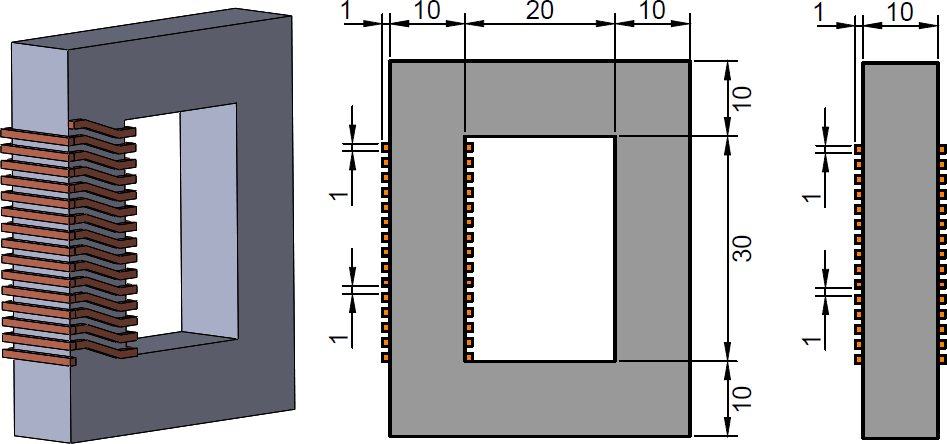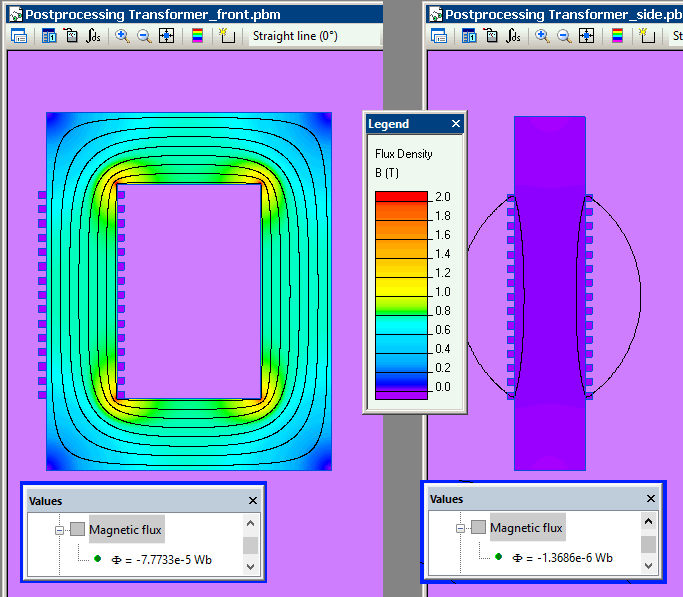Main >> Applications >> Sample problems

# Transformer model 2D vs. 3D

transformer magnetic field, 2D vs 3D FEA finite element analysis

Example courtesy of Sergey Chishko

When modeling an electromechanical device, a designer needs to keep in mind that a 2D problem set introduces an error in a field distribution in comparison with a 3D problem solution. However, a solving process of 2D problems is multiple times faster than of 3D. In this example the main (front) and side views of the transformer are modeled to demonstrate that a contribution of front and end sides of a coil to the total magnetic flux in the core is negligible.

Problem Type
Plane-parallel problem of DC magnetics.

GeometryGiven
All dimensions are in millimeters;
Core has a linear relative permeability of μ = 1000;
Coil conducts constant current I = 5 A.

Obtain and compare the values of magnetic flux over the cross section of a transformer leg holding the coil for transformer's front and side view problems sets.

Solution
To study the impact of end effects on the total magnetic flux in the transformer two models are simulated: front view and side view.
Front-view model (2D) considers only a part of a coil with a current direction "in" and "out" of the plane and neglecting end effects of "front" and "end" coil parts with currents flowing along the plane. Side-view (2D) model considers only "front" and "end" coil parts.
Both models features the same number of turns of the coil and the current.

Results
Front-view model magnetic flux is -77.7 uWb (98.3%).
Side-view model magnetic flux is -1.3 uWb (1.7 %).
Coil end parts produce only 1.7% of the total magnetic flux and could be neglected in most cases.

Magnetic flux in cross sections of the transformer in front and side models: